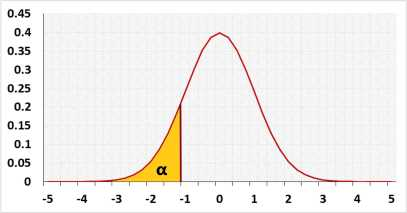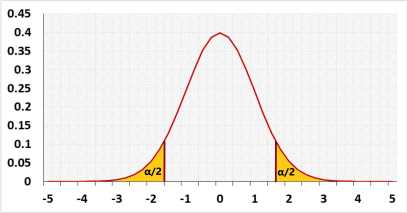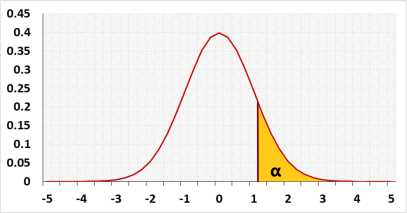# Normal, T - Sample size calculatorPower analysis for the entire model

## Information

Calculate the sample size to gain the required test power and draw a power analysis chart. Use this test for one of the following tests:
One Sample Z-Test
One Sample T-Test
Two Sample Z-Test
Two Sample T-Test (Pooled variance)
Two Sample T-Test (Welch's)

Example:
Linear regression with 4 predictors, α=0.05, power=0.8.
A sample of 85 will identify model with R2=0.13. (or f=0.3873 or f2=0.15)
i.e. the power of a model with a smaller R2 will be lower than 0.8 .

### Distribution## R Code

The following R code should produce the same results